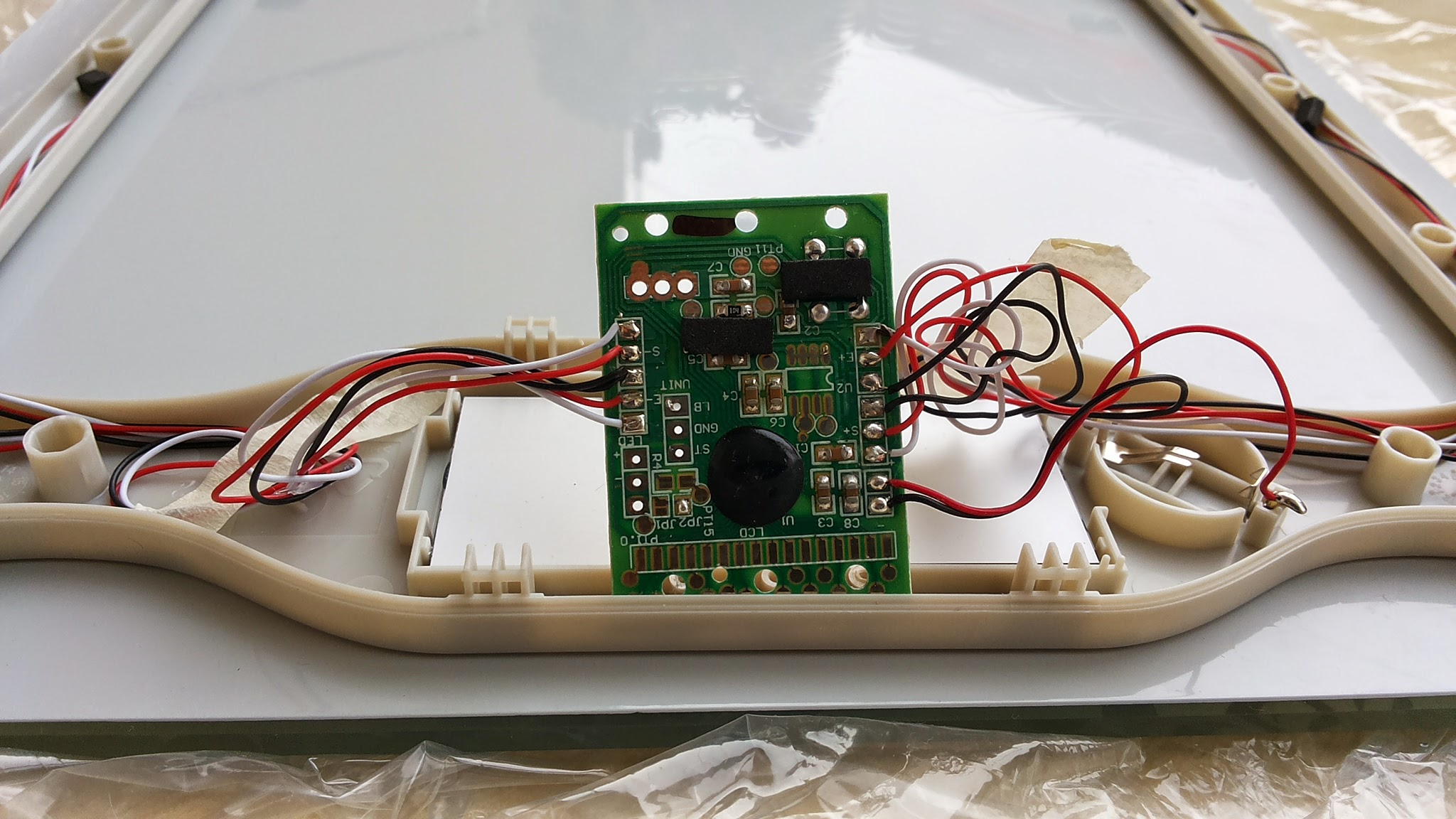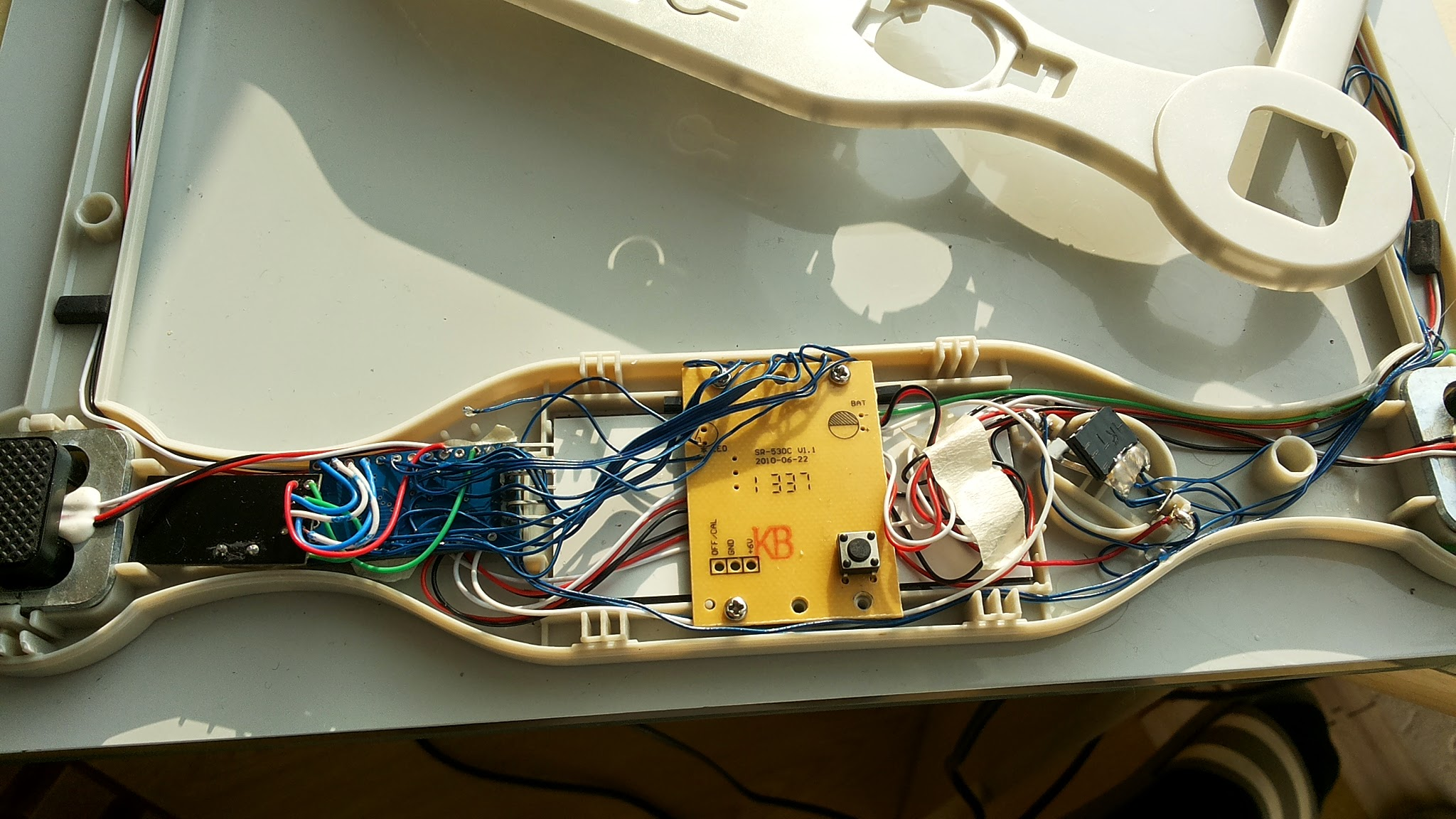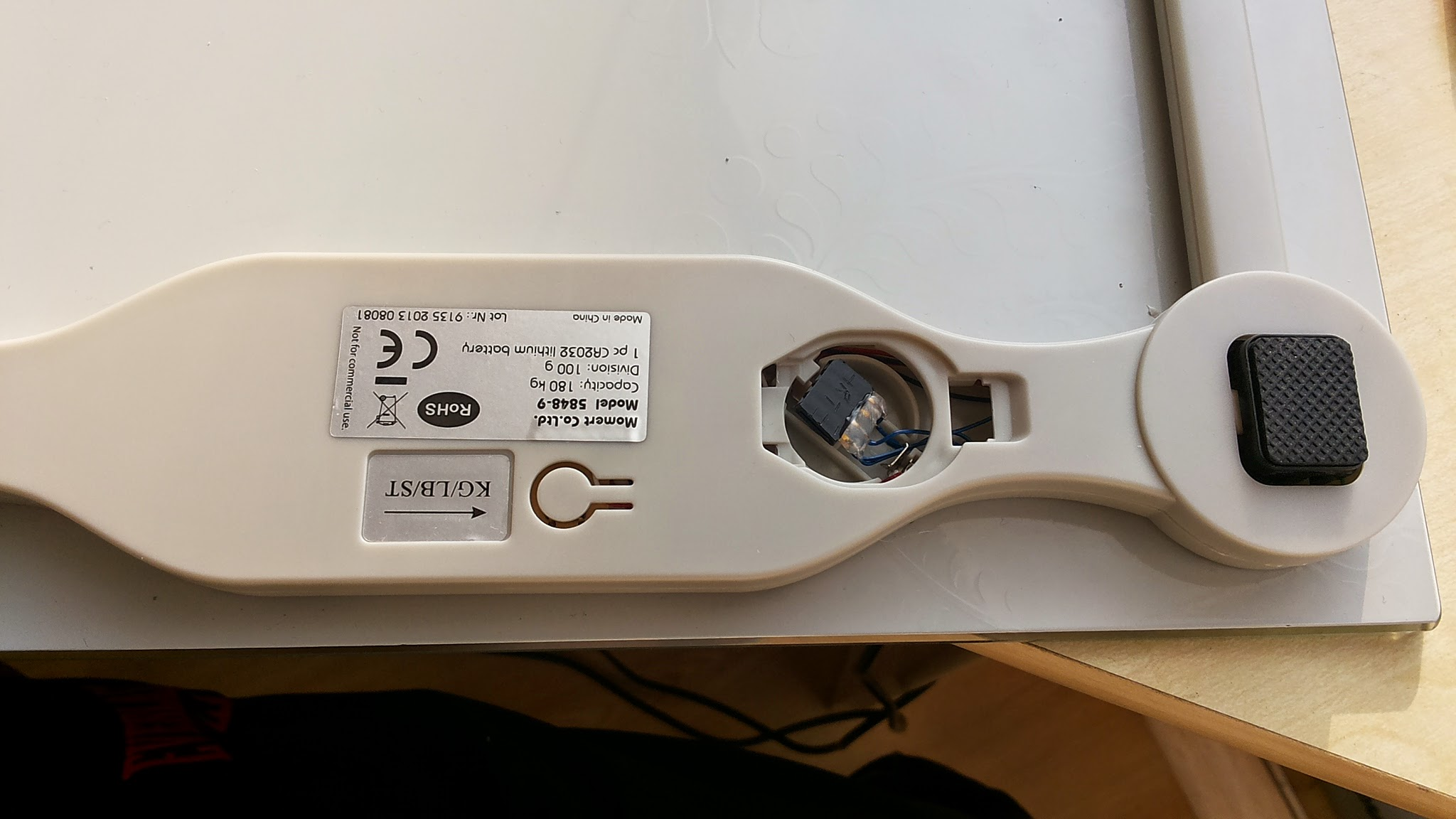# Momert 5848-9 bathroom scale (reading from lcd)

• Pin arrangement the same as on IKEA Grundtal scales http://troels.leegaard.org/misc/grundtal20047/grundtal20047.pdf

Putting 3V to each lcd pin you can figure out what is each pin for. I used 13 lcd pins from 16. https://www.youtube.com/watch?v=yGe3wtwzqf0

Some photosCODE:
#include <Sleep_n0m1.h>
#include <SPI.h>
#include <EEPROM.h>
#include <RF24.h>
#include <Sensor.h>

``````	#define DIGITAL_INPUT_SENSOR 3   // The digital input you attached your motion sensor.  (Only 2 and 3 generates interrupt!)
#define INTERRUPT DIGITAL_INPUT_SENSOR-2 // Usually the interrupt = pin -2 (on uno/nano anyway)
#define DI_ID 1                              // Id of the sensor child
#define SA_ID 2                              // Id of the sensor child
#define VI_ID 3                              // Id of the sensor child
#define ALL_ID 4                              // Id of the sensor child

#define DEBUG 1

int pa,pb,pc,pd;
int           lcdA=0,lcdB=0,lcdC=0,lcdD=0;
bool          lcd1,lcd2,lcd3,lcd4,lcd5,lcd6,lcd7,lcd8,lcd9;
unsigned int  SAn, SBn, SCn, SDn;
unsigned int  SA, SB, SC, SD;
bool          A, B, C, D;
double weight;
double oldweight=0;

Sensor gw;
Sleep sleep;

//calculate weight
int getDigit(int d)
{
// d=0,1,2,3
int i = 2*d+0;
int j = 2*d+1;

if ( A[i] &&  A[j] && !B[i] &&  B[j] &&  C[i] &&  C[j] &&  D[i]) return 0; //ok
if (!A[i] && !A[j] && !B[i] &&  B[j] && !C[i] &&  C[j] && !D[i]) return 1; //ok
if (!A[i] &&  A[j] &&  B[i] &&  B[j] &&  C[i] && !C[j] &&  D[i]) return 2; //ok
if (!A[i] &&  A[j] &&  B[i] &&  B[j] && !C[i] &&  C[j] &&  D[i]) return 3; //ok
if ( A[i] && !A[j] &&  B[i] &&  B[j] && !C[i] &&  C[j] && !D[i]) return 4; //ok
if ( A[i] &&  A[j] &&  B[i] && !B[j] && !C[i] &&  C[j] &&  D[i]) return 5; //ok
if ( A[i] &&  A[j] &&  B[i] && !B[j] &&  C[i] &&  C[j] &&  D[i]) return 6; //ok
if (!A[i] &&  A[j] && !B[i] &&  B[j] && !C[i] &&  C[j] && !D[i]) return 7; //ok
if ( A[i] &&  A[j] &&  B[i] &&  B[j] &&  C[i] &&  C[j] &&  D[i]) return 8; //ok
if ( A[i] &&  A[j] &&  B[i] &&  B[j] && !C[i] &&  C[j] &&  D[i]) return 9; //ok
return 0;
}

void setup()
{
gw.begin();

// Send the sketch version information to the gateway and Controller
gw.sendSketchInfo("Weight Meter", "1.0");

pinMode(DIGITAL_INPUT_SENSOR, INPUT);      // sets the motion sensor digital pin as input
// Register all sensors to gw (they will be created as child devices)
gw.sendSensorPresentation(DI_ID, S_WEIGHT);
gw.sendSensorPresentation(SA_ID, S_WEIGHT);
gw.sendSensorPresentation(VI_ID, S_WEIGHT);
gw.sendSensorPresentation(ALL_ID, S_WEIGHT);
}

void loop()
{

getweightnow();

#if DEBUG
Serial.println("Sleeping.");
#endif
delay(400); //delay to allow serial to fully print before sleep
gw.powerDown();
sleep.pwrDownMode(); //set sleep mode
sleep.sleepInterrupt(INTERRUPT,CHANGE);
}

void getweightnow() {

// waiting for display (interruption will continue from here)
#if DEBUG
Serial.println("Stepped on scale");
#endif

// make sure that LCD is active

// starts measuring
#if DEBUG
Serial.println("Mesuring");
#endif

SAn = SBn = SCn = SDn = 0;
for (int i=0; i<9; i++)
SA[i] = SB[i] = SC[i] = SD[i] = 0;
int i=0;
//run while backplanes are active
while ((lcdA!=0 || lcdB!=0 || lcdC!=0 || lcdD!=0)) {

// decode A, B, C and D signals to -1, 0, 1
pa = 0;
if (lcdA < 300) pa=-1; else if (lcdA > 600) pa=1;
pb = 0;
if (lcdB < 300) pb=-1; else if (lcdB > 600) pb=1;
pc = 0;
if (lcdC < 300) pc=-1; else if (lcdC > 600) pc=1;
pd = 0;
if (lcdD < 300) pd=-1; else if (lcdD > 600) pd=1;
lcdA = pa; lcdB = pb; lcdC = pc; lcdD = pd;

if (lcdA!=0 && lcdB==0 && lcdC==0 && lcdD==0 ||
lcdA==0 && lcdB!=0 && lcdC==0 && lcdD==0 ||
lcdA==0 && lcdB==0 && lcdC!=0 && lcdD==0 ||
lcdA==0 && lcdB==0 && lcdC==0 && lcdD!=0)
{
if (lcdA==1 || lcdB==1 || lcdC==1 || lcdD==1)
{
lcd1 = 1-lcd1;
lcd2 = 1-lcd2;
lcd3 = 1-lcd3;
lcd4 = 1-lcd4;
lcd5 = 1-lcd5;
lcd6 = 1-lcd6;
lcd7 = 1-lcd7;
lcd8 = 1-lcd8;
lcd9 = 1-lcd9;
}

if (lcdA != 0)
{
SA += lcd1;
SA += lcd2;
SA += lcd3;
SA += lcd4;
SA += lcd5;
SA += lcd6;
SA += lcd7;
SA += lcd8;
SA += lcd9;
SAn++;
}
if (lcdB != 0)
{
SB += lcd1;
SB += lcd2;
SB += lcd3;
SB += lcd4;
SB += lcd5;
SB += lcd6;
SB += lcd7;
SB += lcd8;
SB += lcd9;
SBn++;
}
if (lcdC!=0)
{
SC += lcd1;
SC += lcd2;
SC += lcd3;
SC += lcd4;
SC += lcd5;
SC += lcd6;
SC += lcd7;
SC += lcd8;
SC += lcd9;
SCn++;
}
if (lcdD!=0)
{
SD += lcd1;
SD += lcd2;
SD += lcd3;
SD += lcd4;
SD += lcd5;
SD += lcd6;
SD += lcd7;
SD += lcd8;
SD += lcd9;
SDn++;
}

i++;
}

if (lcdA==-1 && lcdB==-1 && lcdC==-1  && lcdD==-1 && lcd9==0 && (SA+SA+SA+SA+SA+SA+SA+SA+SA>0)) {

// measuring is done
#if DEBUG
Serial.println("Done");
#endif

// finding the average of signals values during measuring period
// not 100% correct, but the fastest way I could think of
for (int i=0;i<9;i++)
{
A[i] = (1.0 * SA[i] / SAn >0.5);
B[i] = (1.0 * SB[i] / SBn >0.5);
C[i] = (1.0 * SC[i] / SCn >0.5);
D[i] = (1.0 * SD[i] / SDn >0.5);
}

// decoding
float weight = 100.0*getDigit(0) + 10.0*getDigit(1) + 1.0*getDigit(2) + 0.1*getDigit(3);

#if DEBUG
Serial.print("Weight is: ");
Serial.println(weight);
#endif
if (weight != oldweight) {
gw.sendVariable(DI_ID, V_WEIGHT, weight,3);               // Send volume value to gw
#if DEBUG
Serial.println("Sent");
#endif
oldweight = weight;
}
break;

} //end of if
} //end of while

}
``````

And now is the question S_WEIGHT sensor-type are working on MySensors Arduino Library (v1.3) and VERA Lite as controller. Or do i need *Sensor1.json *Sensor1.xml files for it working? Because when i included scales node to Vera and restarted Luup, after that all nodes stoped working. Only restoring VERA from backup are helped.

• Ohh.. how cool is this! Hacking a scale!

No weight device-files exists for vera yet. Would be great if you created one. There might be one available for the rfxtrx plugin that you could use.

• Wild!
How will you use this? (Its not apparent to me this late at night)

• @ServiceXp said:

Wild!
How will you use this? (Its not apparent to me this late at night )

Every morning, when you stepped on scale, your weight will be sended to Vera, and stored at Datamine Graphing and Logging Plugin.

• Vera Plugin ScaleSensor
ScaleSensor.zip

• Cool thanks.

I'll add it on the next master update.

• Pushed the new scale device files.

• @derrij - Amazing work working this out.
I had this same idea back in about Feb this year...my approach was to hack it the same way as you have done, but i also knew i didnt have the skills ( or time) to work this out...really cool!!!

I think your work/technique has a lot of value in "mySensoring" other devices with LCD/LED digit displays. Eg: A thermostat/control panel built into portable gas heaters- so as to read the display digits.

Related....and cool too, just not as cool...
Not long after there was a bargain on these http://www.withings.com/eu/ws-30.html - Abouit \$40aud so i bought these instead...No they dont hook into mySensors, or Vera...but they are Web-enabled and can work out who the family member standing on the scales...and also tell you if its going to rain that day.

• Wow, i did not do this to my digital weight scale. You did a great job here

1

9

5

8

1

7

9

1